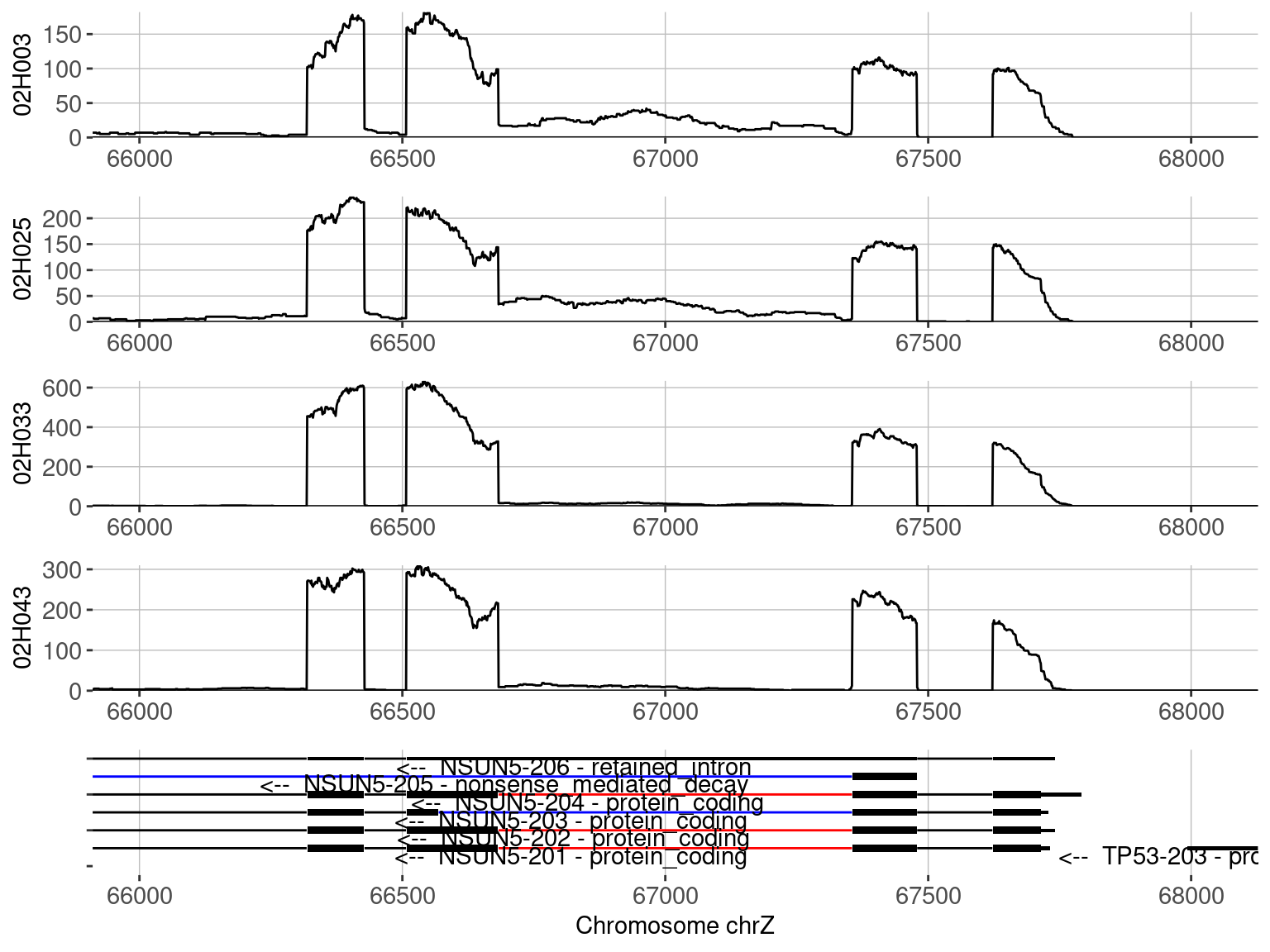# (0) Important announcement

NxtIRFcore will no longer be supported after Bioconductor version 3.16. Its full functionality (plus heaps more) is replaced by SpliceWiz which will be available on Bioconductor version 3.16 onwards.

# (1) Installation and Quick-Start

This section provides instructions for installation and a quick working example to demonstrate the important functions of NxtIRF. NxtIRFcore is the command line utility for NxtIRF.

For detailed explanations of each step shown here, refer to chapter 2: “Explaining the NxtIRF workflow” in this vignette. For a list of ready-made “recipes” for typical-use NxtIRF in real datasets, refer to chapter 3: “NxtIRF cookbook”

### Installation

To install NxtIRFcore, start R (version “4.1”) and enter:

``````if (!requireNamespace("BiocManager", quietly = TRUE))
install.packages("BiocManager")

BiocManager::install("NxtIRFcore")``````

(Optional) For MacOS users, make sure OpenMP libraries are installed correctly. We recommend users follow this guide, but the quickest way to get started is to install `libomp` via brew:

``brew install libomp``

``````library(NxtIRFcore)

### Building the NxtIRF reference

A NxtIRF reference requires a genome FASTA file (containing genome nucleotide sequences) and a gene annotation GTF file (preferably from Ensembl or Gencode).

NxtIRF provides an example genome and gene annotation which can be accessed via the NxtIRFdata package installed with NxtIRF:

``````# Provides the path to the example genome:
chrZ_genome()
#>  "/home/biocbuild/bbs-3.16-bioc/R/library/NxtIRFdata/extdata/genome.fa"

# Provides the path to the example gene annotation:
chrZ_gtf()
#>  "/home/biocbuild/bbs-3.16-bioc/R/library/NxtIRFdata/extdata/transcripts.gtf"``````

Using these two files, we construct a NxtIRF reference as follows:

``````ref_path = file.path(tempdir(), "Reference")
BuildReference(
reference_path = ref_path,
fasta = chrZ_genome(),
gtf = chrZ_gtf()
)``````

### Running IRFinder

NxtIRF provides an example set of 6 BAM files to demonstrate its use via this vignette.

Firstly, retrieve the BAM files from ExperimentHub using the NxtIRF helper function `NxtIRF_example_bams()`. This makes a copy of the BAM files to the temporary directory:

``````bams = NxtIRF_example_bams()
bams
#>   sample                       path
#> 1 02H003 /tmp/RtmpcHmrJB/02H003.bam
#> 2 02H025 /tmp/RtmpcHmrJB/02H025.bam
#> 3 02H026 /tmp/RtmpcHmrJB/02H026.bam
#> 4 02H033 /tmp/RtmpcHmrJB/02H033.bam
#> 5 02H043 /tmp/RtmpcHmrJB/02H043.bam
#> 6 02H046 /tmp/RtmpcHmrJB/02H046.bam``````

Finally, run NxtIRF/IRFinder as follows:

``````irf_path = file.path(tempdir(), "IRFinder_output")
IRFinder(
bamfiles = bams\$path,
sample_names = bams\$sample,
reference_path = ref_path,
output_path = irf_path
)``````

### Collate individual IRFinder runs to build a NxtIRF Experiment

First, collate the IRFinder output files using the helper function `Find_IRFinder_Output()`

``expr = Find_IRFinder_Output(irf_path)``

This creates a 3-column data frame with sample name, IRFinder gzipped text output, and COV files. Compile these output files into a single experiment:

``````nxtirf_path = file.path(tempdir(), "NxtIRF_output")
CollateData(
Experiment = expr,
reference_path = ref_path,
output_path = nxtirf_path
)``````

### Importing the collated data as a NxtSE object:

The `NxtSE` is a data structure that inherits `SummarizedExperiment`

``se = MakeSE(nxtirf_path)``

### Set some experimental conditions:

``````colData(se)\$condition = rep(c("A", "B"), each = 3)
colData(se)\$batch = rep(c("K", "L", "M"), 2)``````

### Perform differential alternative splicing

The code below will contrast condition:B in respect to condition:A

``````# Requires limma to be installed:
require("limma")
res_limma = limma_ASE(
se = se,
test_factor = "condition",
test_nom = "B",
test_denom = "A",
)

# Requires DESeq2 to be installed:
require("DESeq2")
res_deseq = DESeq_ASE(
se = se,
test_factor = "condition",
test_nom = "B",
test_denom = "A",
)

# Requires DoubleExpSeq to be installed:
require("DoubleExpSeq")
res_DES = DoubleExpSeq_ASE(
se = se,
test_factor = "condition",
test_nom = "B",
test_denom = "A",
)``````

### Visualise a Coverage plot of a differential IR event:

Filter by visibly-different events:

``res_limma.filtered = subset(res_limma, abs(AvgPSI_A - AvgPSI_B) > 0.05)``

Plot individual samples:

``````p = Plot_Coverage(
se = se,
Event = res_limma.filtered\$EventName,
tracks = colnames(se)[c(1,2,4,5)],
)
#> Warning: `gather_()` was deprecated in tidyr 1.2.0.
#> ℹ The deprecated feature was likely used in the plotly package.
#>   Please report the issue at <https://github.com/plotly/plotly.R/issues>.
as_egg_ggplot(p)``````Display the plotly interactive version of the coverage plot (not shown here)

``````# Running this will display an interactive plot
p\$final_plot``````

Plot by condition:

``````p = Plot_Coverage(
se = se,
Event = res_limma.filtered\$EventName,
tracks = c("A", "B"),
condition = "condition",
stack_tracks = TRUE,
t_test = TRUE,
)
as_egg_ggplot(p)
#> Warning: Removed 270 row(s) containing missing values (geom_path).``````